### 太意外！非农连传三大坏消息 美元“飞流直下”、黄金美股“一柱擎天”4月份的美国就业情况非常令人失望，非农就业人数增加了26.6万人，远低于预期，失业率上升至6.1%，而可用劳动力的短缺加剧。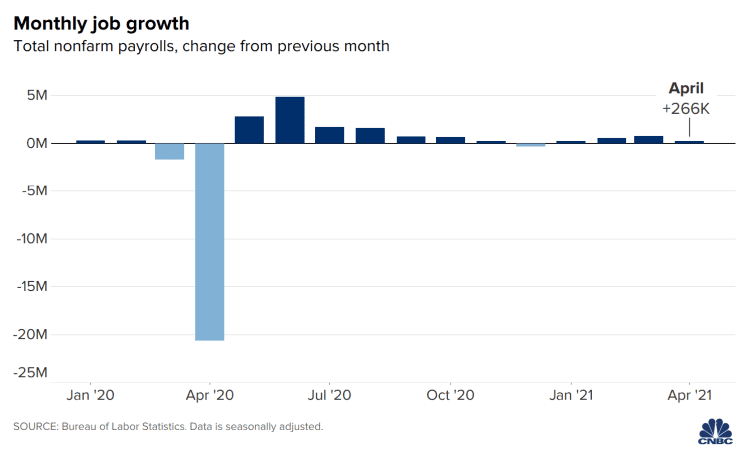500)this.width=500 align=center hspace=10 vspace=10 rel=nofollow/>

500)this.width=500 align=center hspace=10 vspace=10 rel=nofollow/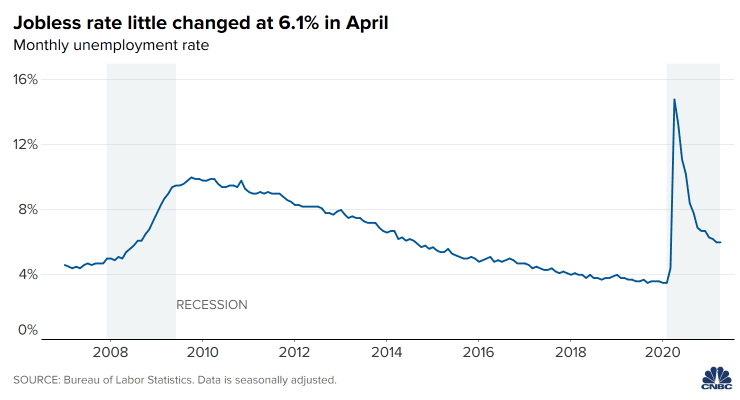500)this.width=500 align=center hspace=10 vspace=10 rel=nofollow/>

500)this.width=500 align=center hspace=10 vspace=10 rel=nofollow/

“我们增加工资。我们大约有三家不同的招聘机构在不断地寻找人才。其他的餐馆老板则在附近走动，发传单。我们社区的英雄是那些为你我工作的人。”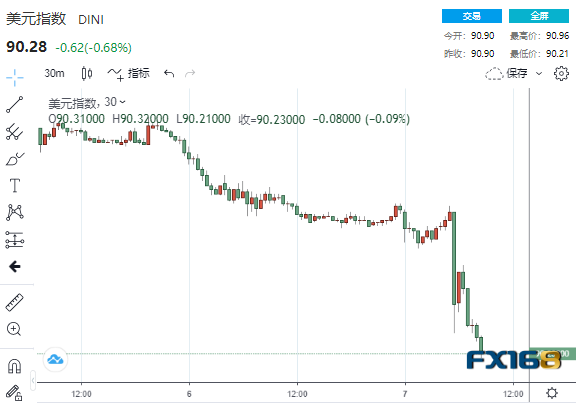500)this.width=500 align=center hspace=10 vspace=10 rel=nofollow/>

500)this.width=500 align=center hspace=10 vspace=10 rel=nofollow/

Schlossberg补充称，这也意味着美国利率将在相当长一段时间内维持在超低水准，将令美元承压。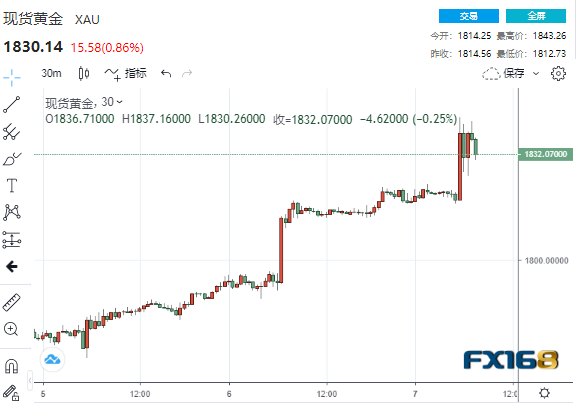500)this.width=500 align=center hspace=10 vspace=10 rel=nofollow/>

500)this.width=500 align=center hspace=10 vspace=10 rel=nofollow/

Blue Line Futures首席市场策略师Phillip Streible表示，由于“(就业)数据完全不达预期”，收益率将暂时压缩，美元指数也跌破支撑位，令金价上扬。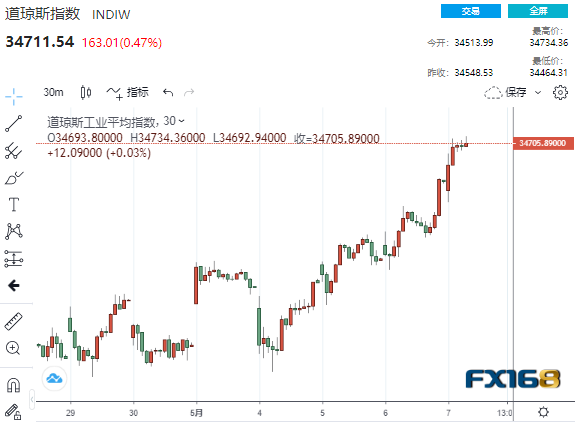500)this.width=500 align=center hspace=10 vspace=10 rel=nofollow/>

500)this.width=500 align=center hspace=10 vspace=10 rel=nofollow/【Kitco黄金调查】唱多声高涨！黄金冲破1800料点燃大涨导火索 市场看涨情绪爆棚

【黄金收盘】非农意外爆冷、美联储加息预期降温 黄金本周大涨62美元、创6个月最大涨幅